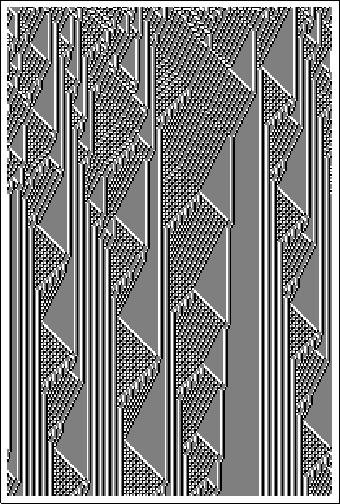#Wolfram Summer School

# Alumni# Chiara Basile

Summer School

Class of 2009

## Bio

Chiara Basile is a PhD student in mathematical physics at the University of Bologna, Italy. Her research ranges from theoretical questions about entropy and information to applications to problems of classification of symbolic sequences of almost any kind: literary texts, medical reports, cellular automata, etc. She has a passion for foreign cultures and languages, walking, improv theater, and Mathematica.

## Project: Exploring CA Rule Spaces by Means of Data Compression

This project is aimed at the automatic exploration of the space of ECA rules and other slightly more complex (larger range / more colors / 2D) CA rule spaces. The goal of this exploration is to find interesting evolutions for CA; some of the questions to try to answer are the following:

• Which simple initial conditions give rise to different, interesting evolutions for a given rule?
• What fraction of simple initial conditions (up to some threshold) give rise to essentially the same evolution?
• What are the interesting time steps, i.e. the ones for which the evolution changes sensibly?
• Given a non-elementary CA rule, which ECA rule has the most similar evolution?

The main tool this project will use to mine rule spaces is data compression, which has shown itself to be a very powerful instrument for pattern recognition and has often been used to solve problems of symbolic sequence classification/clustering. This project will concentrate on Lempel-Ziv-like (L2) data compressors, which have been proved to be universally optimal, i.e. they compress an infinite string to the entropy of the information source that generated it. Compressors are therefore good candidates as approximators of the complexity (or entropy, or information content) of strings. The Compress function in Mathematica, which has already been used to cluster CA evolutions, is a compression algorithm based on a mix of an LZ-like compression scheme and Huffman coding, called the Lempel-Ziv-Welch (LZW) algorithm; this same algorithm is at the base of the widespread gzip data compression software.

The evolution of a 1D CA can be represented as a 2D matrix (this is what actually happens with the CellularAutomaton function in Mathematica); on the other hand, LZ-like compressors work by sequentially reading a unidimensional, linear sequence. Therefore one has to choose exactly what to feed the data compressor, being aware that even if the compressor is indeed universally optimal, different choices about how to “read” the CA matrix can give rise to different entropy measures, as we are forced to consider finite sequences instead of infinite ones. Indeed, different directions in the CA evolution matrix can correspond to different types of similarities: for example, complex rules with localized structures moving on a periodic background (like ECA rule 110) usually have more repetitions along the columns than along rows, whereas rule 30 has many triangular (bidimensional) structures that cannot be detected by reading it either along rows or along columns. Therefore, three sequences will be associated with each CA evolution: one obtained reading it sequentially along rows, the second given by a sequential reading along columns, and the third by reading the evolution along the Peano-Hilbert plane-filling curve. The three corresponding compression rates will then be a sort of “fingerprint” of the evolution of that rule from that initial condition, and will be used to compare it to other evolutions.

## Favorite Three-Color Cellular Automaton

Rule 333169794290APPLY NOW